# Praxis I Math: Geometry Chapter Exam

Exam Instructions:

Choose your answers to the questions and click 'Next' to see the next set of questions. You can skip questions if you would like and come back to them later with the yellow "Go To First Skipped Question" button. When you have completed the practice exam, a green submit button will appear. Click it to see your results. Good luck!

### Page 1

#### Question 3 3. What is the line, as shown in the picture, called?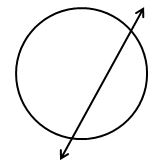### Page 2

#### Question 8 8. What is the area of the right triangle shown below?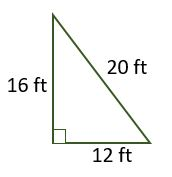#### Question 9 9. In the picture shown below, there are three lines marking each side of the triangle. These lines give the indication that the triangle is: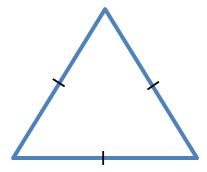#### Question 10 10. What type of triangle is pictured?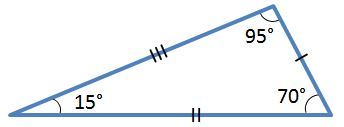### Page 3

#### Question 14 14. What is the area of the pictured rectangle?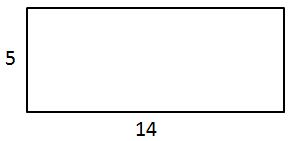#### Question 15 15. If angle a is 66 degrees and angle b is 54 degrees, what is the measure of angle d?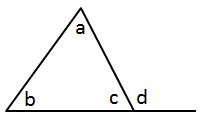### Page 4

#### Question 16 16. If angle a is 60 degrees, what is the measure of angle b?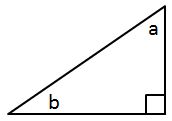#### Question 17 17. The pictured shape is a: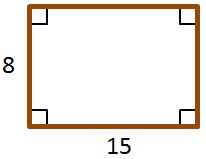#### Question 18 18. The pictured shape is a: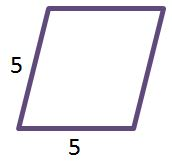### Page 5

#### Question 23 23. In the figure below, Angle 13 = 10y and Angle 11 = 3y + 11. Determine the measure of Angle 16.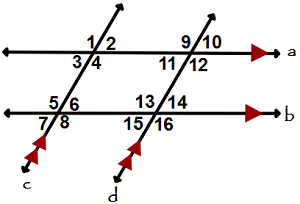#### Question 24 24. In the figure below, Angle 7 = 15x and Angle 10 = 9x + 30. Determine the measure of Angle 10.### Page 6

#### Question 28 28. What is the area of the annulus/doughnut shown below?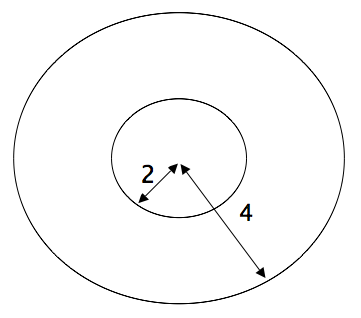#### Question 29 29. In the right triangle pictured below, what is the value of angle x?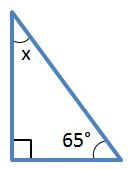#### Praxis I Math: Geometry Chapter Exam Instructions

Choose your answers to the questions and click 'Next' to see the next set of questions. You can skip questions if you would like and come back to them later with the yellow "Go To First Skipped Question" button. When you have completed the practice exam, a green submit button will appear. Click it to see your results. Good luck!

Support